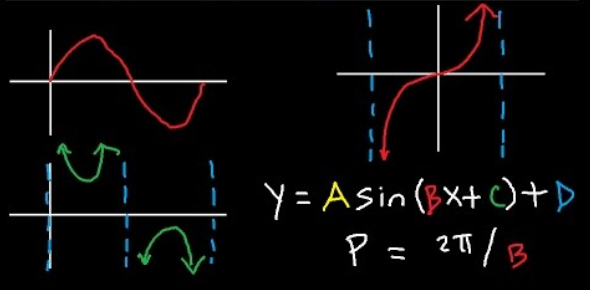# Graphing Trigonometric Functions Quiz! Exam

10 Questions | Attempts: 4873
ShareSettingsBelow are the graphs of the six trigonometric functions: sine, cosine, tangent, cosecant, secant, and cotangent. In this quiz, you will have to identify the equation of a graphed trigonometric function. View the graph and select the correct answer. So, let's try out the quiz. All the best!

• 1.
Select the equation represented by the graph below.
• A.

Y = cos(x)

• B.

Y = sin(x)

• C.

Y = tan(x)

• D.

Y = -sin(x)

• E.

Y = 2cos(x)

• 2.
Select the equation represented by the graph below.
• A.

Y = cos(x)

• B.

Y = sin(x)

• C.

Y = tan(x)

• D.

Y = -sin(x)

• E.

Y = 2cos(x)

• 3.
Select the equation represented by the graph below.
• A.

Y = cos(x)

• B.

Y = sin(x)

• C.

Y = tan(x)

• D.

Y = -sin(x)

• E.

Y = 2cos(x)

• 4.
Select the equation represented by the graph below.
• A.

Y = .5cos(x)

• B.

Y = sec(x)

• C.

Y = csc(x)

• D.

Y = 2sin(x)

• E.

Y = -cos(x)

• 5.
Select the equation represented by the graph below.
• A.

Y = sec(x)

• B.

Y = tan(x)

• C.

Y = -csc(x)

• D.

Y = cot(x)

• E.

Y = sin(x)

• 6.
Select the equation represented by the graph below.
• A.

Y = sec(x)

• B.

Y = tan(x)

• C.

Y = -csc(x)

• D.

Y = cot(x)

• E.

Y = sin(x)

• 7.
Select the equation represented by the graph below.
• A.

Y = -tan(x)

• B.

Y = sec(x)

• C.

Y = 2sin(x)

• D.

Y = -csc(x)

• E.

Y = cot(x)

• 8.
Select the equation represented by the graph below.
• A.

Y = .5cos(x)

• B.

Y = 2sin(x)

• C.

Y = 2cos(x)

• D.

Y = .5sin(x)

• E.

Y = sin(x)

• 9.
Select the equation represented by the graph below.
• A.

Y = .5cos(x)

• B.

Y = 2sin(x)

• C.

Y = 2cos(x)

• D.

Y = .5sin(x)

• E.

Y = sin(x)

• 10.
Any situation that can be modeled with a sine function can also be modeled with a cosine function.
• A.

True

• B.

False

## Related Topics

×

Wait!
Here's an interesting quiz for you.